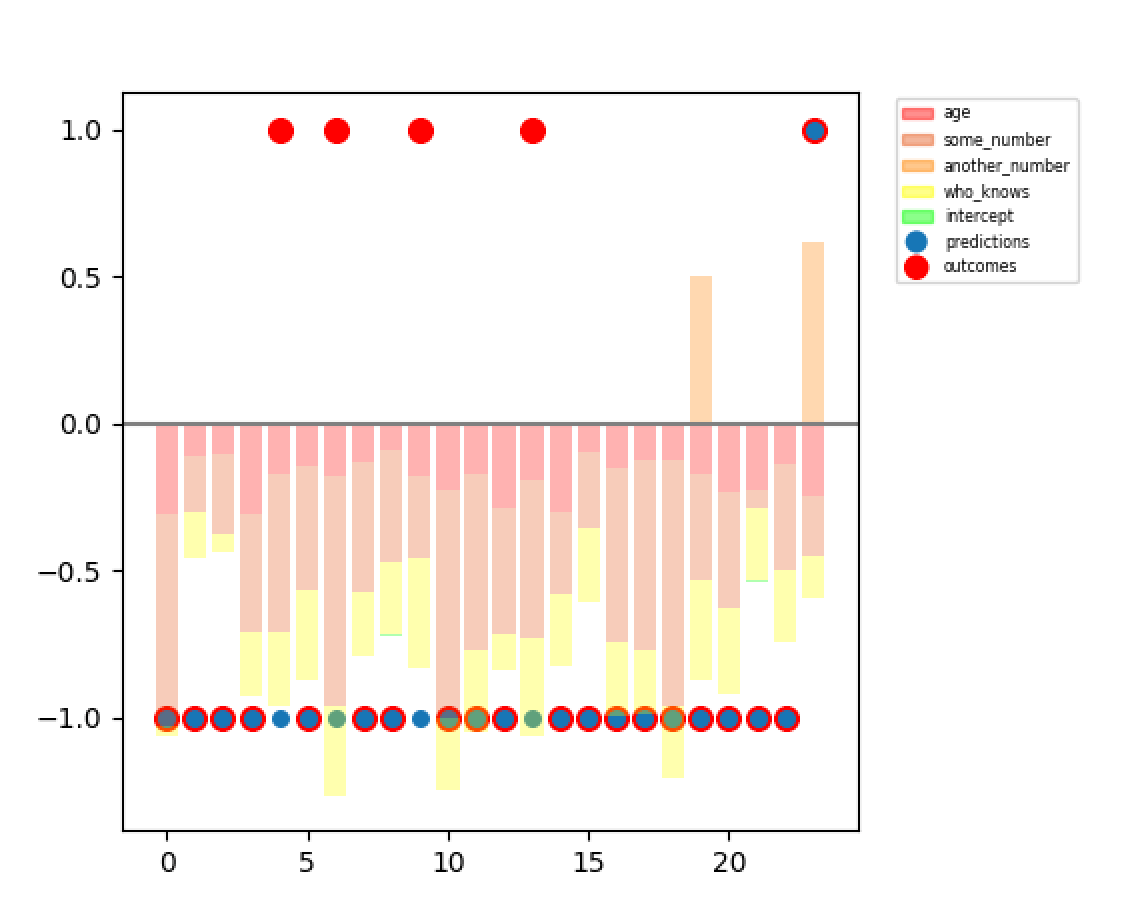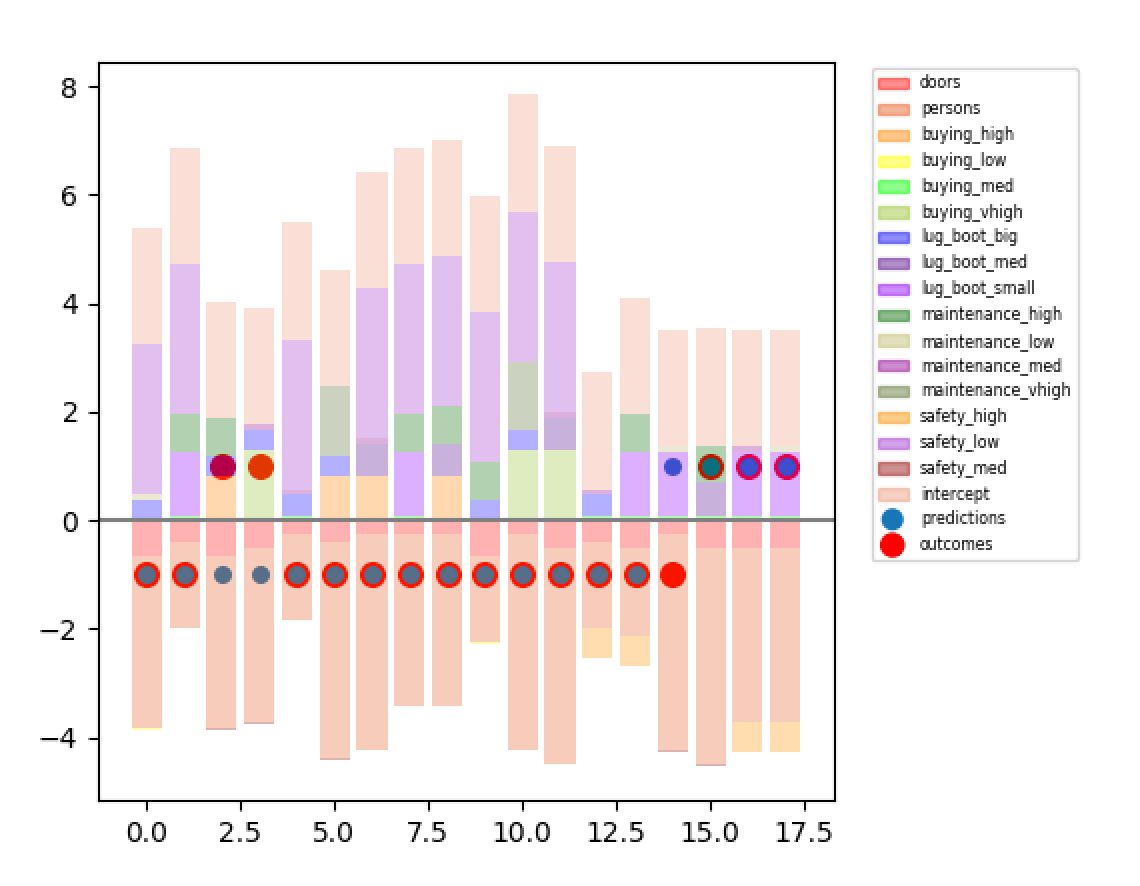# Progress Report on Error Analysis Visualizations: Classification Added!

In September I shared some ideas about visualizing multivariate linear regression in a way that allows SMEs to interpret their data and understand what the models are doing. Then I worked some more on those charts and showed you my progress.

It’s worth noting that up until that point I had only done these visualizations for regression data. I tossed around several different ideas for adapting the method to classification. I decided to step away from the problem for a while, got caught up with other ideas, and ended up stepping away for longer than I initially projected. But this weekend I went ahead and re-orchestrated the visualization to handle binary classification by representing the x-axis of the visualization as the separation boundary between the two classes.

I’ll note before I begin: this approach we’re trying here is not novel. This is, to some degree, what Lime does. Lime does it for much more complicated cases: multiclass classifications, images and text data, et cetera. Lime runs into some of the same limitations we do: it’s hard to do overview error analysis with a tool like this. In fact, Lime analyzes one prediction example at a time. This visualization lets us look at simpler data and analyze feature contribution more examples at a time (maybe 20). Aggregate error analysis visualizations remain a growth area for data science :).

### Example 1: Visualizing Income Data

In order to get the basic thing running, I ran with a no-edge-case example: a classification of income at <=50k or >50k, given a few all-numerical variables. The data labels were not 100% clear, so when I pulled them into a csv I really didn’t know what some of them were (hence the feature names in the legend). That’s OK: I didn’t set out to analyze this particular data. I set out to get the classification visualizations working. So we kept going.If the blue dot appears below the horizontal axis, the model predicted this data to match an income <=50k. If the blue dot appears above the horizontal axis, the model predicted >50k.

The data points appear in no particular order, since we weren’t looking to find a particular trend as makes sense for regression.

Like the regression bars, the bars you see visualize how each feature value, multiplied by its weight, adds up to the model’s prediction. If the bar above the line is taller than the bar below the line, we get an above-the-line prediction. Same for below the line.

As you can see, this model run in particular has a few false <=50k predictions. It doesn’t mean much—I ran this model several times, and that wasn’t always the case.

OK, we got the base case working. Let’s see how the visualization looks in a bit more realistic case—more features, not all numerical, et cetera.

### Example 2: Visualizing Automobile Data

This is a different dataset than the automobile dataset from the last post about these visualizations. We’re classifying here on “evaluation”—I think from a dealership for buying pre-owned cars.Here we had several categorical variables that we needed to one-hot encode. It appears to work, but one thing I’m noticing is this:

For some reason most of the time the bar looks longer in one direction but the prediction is on the other side of the axis. I’m not sure exactly why that is yet. I don’t know if it has something to do with this representation for one-hot encoding or not. I’ll update you if I figure it out.

### Sources:

Census Income 2016: